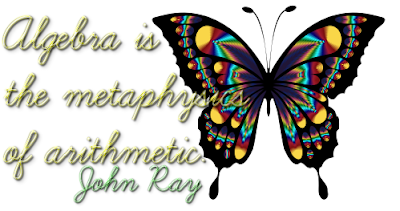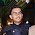## Linear Equations In One Variable

Class 8

#### Q & A based on Class 8 NCERT Chapter

Q1(MCQ): If 3x – 4 (64 – x) = 10, then the value of x is

(a) –266
(b) 133
(c) 66.5
(d) 38

3x - 256 + 4x =10
7x = 10 + 256 = 266
x = 266/7 = 38

Q2: Three consecutive even numbers whose sum is 156 are 51, 52 and 53.
State if the above statement is True or False

Let the three consecutive even numbers be x, x+2, x+4
∴ x + x+ 2 + x+4 = 156
⇒ 3x + 6 = 156
⇒ 3x = 156 - 6 = 150
⇒ x = 150 /  3 = 50
Thus numbers are 50, 51, 52

Q3: Fifteen added to thrice a whole number gives 93. The number is __________.

Q4: x = –12 is the solution of the linear equation 5x –3(2x + 1) = 21+x (True or False)

For x = -12,
LHS = 5(-12) -3[2(-12) + 1] = -60 -3[-26+ 1]  = -60 -3(25) = -60 + 75 = 15
RHS = 21 + (-12) = 21 - 12 = 9
Thus LHS ≠ RHS

Q5: The present age of father is four times the age of his son. After 10 years, age of father will become three times the age of his son. Find their present ages.

Answer: Let the present age of son be x years
∴ The present age of father = 4x years
After 10 years,
Age of son = (x + 10) years
Age of father = (4x + 10) years

According to the given condition
4x + 10 = 3(x + 10)
4x + 10 = 3x + 30
4x – 3x = 30 –10
x = 20
∴ Present age of son = 20 years.
and present age of father = 4x = 4 × 20 = 80 years.

Q6(MCQ): If 8x – 3 = 25 +17x, then x is

(a) a fraction
(b) an integer
(c) a rational number
(d) cannot be solved

Answer: (c) a rational number
8x - 3 = 25 + 17x
On transposing,
8x - 17x = 25 + 3
-9x = 28
x = -28/9  which is a rational number.

Q7(MCQ): The shifting of a number from one side of an equation to other is called

(a) Transposition
(b) Distributivity
(c) Commutativity
(d) Associativity

Q8(MCQ): A linear equation in one variable has

(a) Only one solution
(b) Two solutions
(c) More than two solutions
(d) No solution

Answer: (a) Only one solution

Q9(MCQ): The sum of three consecutive multiples of 7 is 357. Find the smallest multiple.

(a) 112
(b) 126
(c) 119
(d) 116

Let the numbers be x, x+7, x+14
∴ x + x + 7 + x + 14 =  357
⇒ 3x + 21 = 357
⇒ 3x = 357 - 21 = 336
⇒ x  336 / 3 = 112

Q10: The solution of the equation 3x – 4 = 1 – 2 x is _________.

Q11: In a linear equation, the _________ power of the variable appearing in the equation is one.

Q12: Rs. 13500 are to be distributed among Salma, Kiran and Jenifer in such a way that Salma gets Rs. 1000 more than Kiran and Jenifer gets Rs. 500 more than Kiran. Find the money received by Jenifer.

Let Kiran gets = ₹p
Salma gets - ₹p + 1000
Jenifer gets = ₹p + 500
∴ Sum is p + p + 1000 + p + 500 = 13500
⇒ 3p = 13500 - 1500 = 12000
⇒ p = 12000/3 = 4000
∴ Jenifer gets = 4000 + 500 = ₹ 45001.2.